×

×

UTEP / Chemistry / CHEM 2321 / what are the factors that affect boiling point?

# what are the factors that affect boiling point? Description

##### Description: This study goes over things that make be on the test as well as tips and tricks to remember boiling point, melting point, acidity and even oxidation states.
11 Pages 20 Views 5 Unlocks
Reviews

jazz (Rating: )

Cynthia Lugo (Rating: )

Organic Chemistry Exam 2 Study Guide Important Information:

## what are the factors that affect boiling point?Professor’s Email: jsal@utep.edu

Class Website: organic.utep.edu/courses/2324

Factors that Effect Boiling Points:

1. The relative strength of the four intermolecular forces: Ionic > Hydrogen bonding> diploe  dipole> Van der Waals dispersion forces. (These are influenced by the function group present).  2. Boiling points increases as the number of carbons increase.

3. Branching decreases boiling point

Examples of the intermolecular forces affecting boiling point: If you look ionic has the higher  boiling point, while the dipole dipole has the least.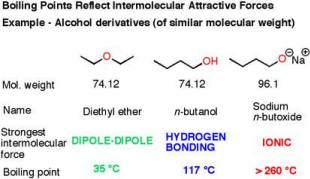## what is the order of intermolecular forces?Now let’s think about if there are no polar function groups such as butane C4H10, the only  forces going on are Van der Waals, so this would have a very low boiling point. So let’s look at  amine and carboxylic acids as well, so you can see the trend. If you want to learn more check out bio 315 exam 1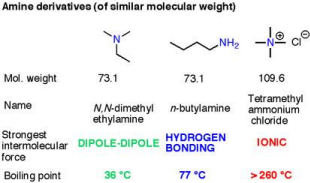Don't forget about the age old question of assentote
If you want to learn more check out unit 6 study guide math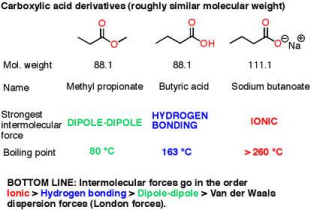## Factors that Effect Melting Points?Now the second factor affecting boiling point is increasing carbons, this also is for the molecular  weight the higher it is, the higher the boiling point.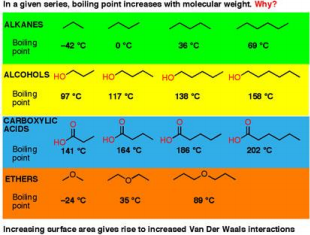As you can see as all of the examples get bigger the boiling points increase dramatically.  Now the final reason symmetry (branching). If something is symmetrical the likely hood of it  lining up and bond is better, when it can’t its boiling point is higher.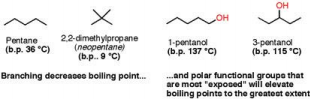We also discuss several other topics like What fields are related to psychology?

If we look at neopentane it is symmetrical so it can stack better, it is highly “branched” so its  boiling point is very low.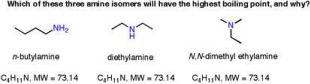If we follow the trends the first one will have the highest (its unbranched, a large number of  carbons, and hydrogen bonding), the second highest will be NH, then N on the right, it is highly  branched which leads to a low boiling point.

Factors that Effect Melting Points:

1. Increases with size and weight. Like boiling points the bigger the molecule the bigger the  melting point. This also includes if a Cl vs. N is on a 5-carbon chain the Cl has a higher weight and  will have a higher melting point.

2. Increase with bonds, this is the same as with boiling point. Ionic > Hydrogen bonding> diploe  dipole> Van der Waals dispersion forces. (These are influenced by the function group present). 3. Melting point decreases with branching.

4. Increases with better crystal packing, if the molecules can fit nicely together, they will resist  melting.

5. Increases with higher rotational symmetry. Rotational symmetry means you can turn the  molecule and it still will look the same.If you want to learn more check out grople

Let’s look at the following three examples for BOILING and MELTING points.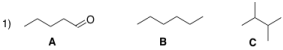Compound A has a strong polarized functional group, an aldehyde. This is creating a strong  molecular dipolar (partial + and – charges). Thus it will form dipole-dipole interactions. This will  have both the higher melting and boiling point.

B and C are alkanes, B is a straight chain while C has lots of branching. In both boiling and  melting points branching decreases the change, so B will have a greater melting and boiling  point than C.

Let’s look at a few other examples: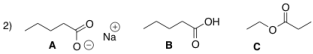If you want to learn more check out chem 322

Molecule A has an ion, or ionic. Because of this if we look at the chart it has the strongest bonds,  so it will have the highest melting AND boiling point.

Molecule B has an OH group, this hydrogen bonding, and will have the second highest of the  boiling and melting points.

Molecule C does have polar functional group, but none of the oxygens bear an acidic proton so  they only form weak dipole-dipole interactions.

Factors that Effect Acidity:

1. Charge. Acidity increases with increasing positive charge on an atom.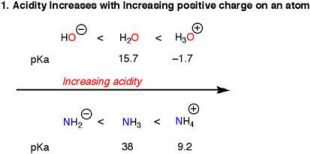2. The role of the atom. This can be confusing because acidity increases as we go across a ROW in  the periodic table, electronegativity plays a role in this. However, going down the periodic table  increases acidity. Think of this because as the molecule gets bigger there is size mismatch. –3. Resonance, if the conjugate base is stable, the acidity will increase.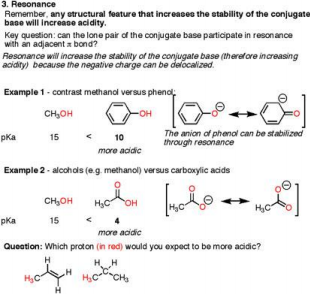The answer to previous question would be the first molecule because it will have a resonance structure.

4. Inductive Effects – electronegative atoms draw negative charges to themselves, this leads to a  stable conjugate base.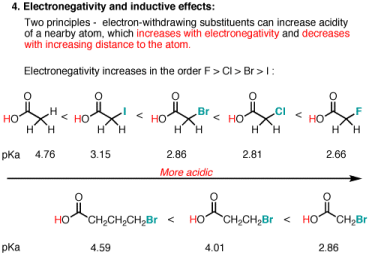As you can see when the Br in the second example is CLOSER to the oxygen the compound is  more acidic because the Br can draw in the oxygen.

5. Orbitals. sp>sp2>sp3 As the orbital is bigger the compound is less acidic.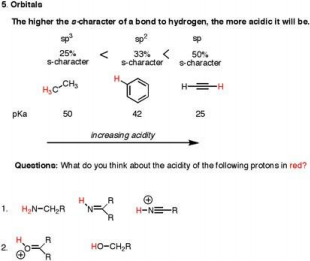In Number 1 the first compound will have the lowest acidity followed by the second from the  left, the then the compound on the right.

Now for the second problem we need to think about charges the first compound will be more  acidic because it bears a positive charge.

Nucleophile – donate electrons

To find the number of moles an reaction will be at a certain point use the following equation(this can be  used for Molarity as well):

10−��������+�������� = �� =��2

Example: p Ka 15.1

H

N1

H

O

+N2 H

p Ka 10.8 N3+

H H

+

H

N4-

O

(��1 − ��)(��2 − ��)

0.5 mole 500 mole

1. Given the number of moles of reactants underneath the reaction to the nearest tenth, how many moles  of the first reactant will be left at equilibrium?

a. 0.1 b. 0.2 c. 0.3 d. 0.4 e. not  a.-d.

We have the pKa forward and backward so 10-15.1+10.8=5.0E-5=K

10−��������+�������� = 5.0E − 5 =��2

(.5 − ��)(500 − ��)

From here you should plug in the x numbers and if it returns the K of 5.0E-5, it is your  number. The answer ends up being A.

How to calculate density by specific gravity:

1. Find the molecular weight of the compound.

2. Divide by the total number of atom in the molecule

3. Divide by water’s specific gravity (18/3 =6). Then round to two significant figures.  Example: C7H6O

The molecular weight will be 12(Carbon’s weight) times 7 + 16(oxygen’s weight) time 1  + 1(hydrogen’s weight) x 6. This equals 106, now divide that by the total number of  atoms in the molecule which is 14. This equals 7.57, now divide that by water’s specific  gravity which is 6, this gives a final density of 1.26 and rounded to 1.3.

If the number is less than 1, the compound will float.

If the number is equal to 1 (or close to 1) the compound will emulsify.

If the number is greater than 1 the compound will sink.

Addition reactions is a reaction where a molecule reacts with another molecule having one or more  multiple covalent bonds, so it forms a molecule whose molecular mass is the sum of the molecular  masses of the reacting molecules. Examples: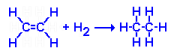In both of these reactions something was added, the double bond goes away because of the addition.

Substitution reactions are reactions in which an atom (or group of atoms) in a molecule is replaced by  another atom or group of atoms. Examples:

In this reaction Br replaces the H.  In this reaction the OH is replaced by I. If you look just  because two atoms were removed it doesn’t mean two must be replaced, the Iodine was place to sub  for the OH.

Elimination reactions are reactions where a multiple covalent bond is formed in a molecule by the  removal of another usually smaller molecule. Examples:

In this reaction the acid pronates the OH group and this  leads to its elimination as water.

In this reaction both the H and Br are eliminated to form HBr  and the carbon goes from a single to triple bond.

Oxidation and Reduction:

So a reductive will result in a net increase in the number of C-H bonds, or a decrease in number of C-O  bonds (this applies for C-Cl, C-Br, etc.)

An oxidation will result in net decrease in the number of C-H bonds or an increase in the number of C-O  bonds (or C-Cl, C-Br etc.)

Anti, Syn, Exo, and Endo:

Exo and Endo are only used with the methyl is on the two largest bridged.

Endo looks like: As you can see there is a bridge formed when you line the bridgehead together, and  if the methyl is pointing downward in relation to the cup it is Endo. Please note this only applies if  the bridgehead’s hydrogens are cis!

Exo: Is up in relation to the bridge, this again only is true if the bridgehead hydrogens are cis.

Syn and Anti are used when the methyl is on the smallest bridge, this is usually on a bridge that is in  the middle of the bridgehead. Syn means the methyl points to the largest bridge, Anti means the  methyl points away from the largest bridge.

Cis and trans are used when the methyl is on the bridgehead.

Endo Rule (Alder Rule)- As the pi bonds of the electron withdrawing group approach the C2 and C3  atoms of the diene, the proximity of the electron withdrawing group to these carbons results in a  secondary overlap between orbitals of the carbons and orbitals of the electron withdrawing group.

E or Z nomenclature

Z and E is a general system to describe cis and trans relationships. The E-Z method of naming is  based on the Cahn-Ingold Prelog system of substituent priorities.

To determine whether E or Z assign each group bonded to doubled bonded carbons are priority  number. Assign groups to the left a 1 and 2 and right 1 and 2. Assign 1 to group with the atom  having a higher atomic number.

As you can see in the above image Z makes a “boat” and E looks more like a “chair” if you don’t look  at the hydrogens and just look at the methyls. So if #1 groups are on the same side it is Z isomer, if  #1 groups are on opposite sides it is E isomer.

R or S

R or S can be tricky, but you don’t have to redraw it every time to do it. Instead leave the hydrogen  where it is and still rank the others. You will end up either going counterclockwise or clockwise. This  table will help:

So if you are looking at the molecule backwards, if you redrew to where the fourth ranked was in the  back it would be opposite of what you originally thought.

Page ExpiredIt looks like your free minutes have expired! Lucky for you we have all the content you need, just sign up here
References: